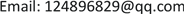MEMS惯性传感器，室内定位，零速区间，卡尔曼滤波, MEMS Inertial Sensor Indoor Positioning Zero Velocity Interval Kalman Filter1. 引言

MEMS IMU以其自主性强、输出频率高、短时精度高、低成本、小体积等优点成为室内导航的理想设备。基于MEMS IMU的室内导航系统中最常用的结构是Foxlin提出的鞋绑式结构  ；文献  将惯性传感器放在腰上，其数据平稳动态性弱，但是行走过程中零速区间不明显，对积累误差的修正较为复杂。零速修正(Zero Velocity Update, ZUPT)和零角速度修正(Zero Angular Rate Update, ZARU)是惯导系统中常用的修正算法，其中最为关键的就是零速区间的检测。

2. MEMS惯性传感器室内定位系统原理

MEMS惯性传感器室内定位系统主要由三部分组成，分别为导航算法部分，零速检测部分以及误差修正部分。

3. 适应于多步态零速区间检测算法

3.1. 判断阈值选取

Selection of threshold parameter

t h a min 和 t h a max 为比力模值的阈值， t h σ 为比力方差的阈值， t h w 为加速度模值的阈值。

3.2. 判断当前运动状态

f i | = f x i 2 + f y i 2 + f z i 2 (1)

f max − f min < 3   g 是正常行走的范围；

3   g < f max − f min < 4   g 是快速行走的范围；

4   g < f max − f min < 7   g 是上下楼梯的范围；

f max − f min > 7   g 是大踏步或跳跃的范围。

3.3. 零速区间检测

condition 1 = { 1 0 t h a min < | f k | < t h a max else (2)

σ f k 2 = 1 2 m + 1 ∑ j = k − m k + m ( f j − f ¯ k ) 2 (3)

condition 2 = { 1 0 σ f k < t h σ else (4)

condition 3 = { 1 0 | w k | < t h w else (5)

4. 零速修正滤波算法

X = [ δ φ ε b δ p n δ v n ∇ b ] T (6)

ε b 表示三个轴向的陀螺漂移；

δ p 表示导航坐标系下导航解算的位置误差；

δ v n 表示导航坐标系下导航解算的速度误差；

∇ b 表示三个轴向的加速度误差。

Z k = [ δ v n ] T = [ v n ] T − [ 0 0 0 ] T (7)

{ X ˙ ( t ) = F ( t ) X ( t ) + W ( t ) Z k = H k X k + V k (8)

H k = [ 0 3 × 3 I 3 × 3 0 3 × 3 0 3 × 3 0 3 × 3 0 3 × 3 0 3 × 3 0 3 × 3 I 3 × 3 0 3 × 3 ] (9)

5. 定位实验验证5.1. 硬件介绍

Hardware performance inde

N表示该项没有具体指标。

5.2. 平面行走实验

Contradistinction of plane walking result in two way

5.3. 三维上下楼实验

Contradistinction of stair walking result in two way

6. 结论